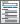Home  /  Products  /  Features  /  Bayesian model averaging

## Bayesian model averaging New

Perform Bayesian model averaging with the bma suite to account for model uncertainty in your analysis. Perform model choice, inference, and prediction. Identify influential models and important predictors. Explore model complexity, model fit, and predictive performance. Perform sensitivity analysis to the assumptions about importance of models and predictors. Generate predictions. And much more.

Learn about Bayesian model averaging and see examples of Bayesian model averaging features.

Watch Bayesian model averaging.

Bayesian model averaging (BMA) for linear regressionBMA posterior summaries

Simulating posterior distributions of model parameters• Analytical posterior distributions
• MCMC-based posterior distributions

Model and variable-inclusion summary• Models ranked by PMP
• Highest probability model (HPM)
• Median probability model (MPM)
• Models containing specific predictors

Posterior distributions of regression coefficientsModel-size distribution summariesBMA plots

• Doppelhofer—Weeks measure
• Ley—Steel type 1 measure
• Ley—Steel type 2 measure
• Yule's Q measure
• Modified Yule's Q measure
• Analytical LPS
• Frequency-based LPS
• LPS summaries
• Entropy
• Analytical posterior predictive means and standard deviations with fixed g
• MCMC-sample posterior predictive summaries: mean, median, standard deviation, and credible intervals
• Predictions of simulated outcome
• Replicates of simulated outcome• Log predictive-score

Postestimation Selector• View and run all postestimation features for your command
• Automatically updated as estimation commands are run

Posterior summariesMCSE estimation methodsModel goodness of fit

Tools to check MCMC convergence

• Diagnostic plots in compact form
• Trace plots
• Autocorrelation plots
• Histograms
• Density plots
• Cumulative sum plots
• Bivariate scatterplots
• Produce any of the above for parameters or functions of parameters
• Multiple separate graphs or multiple plots on one graph
• Pause between multiple graphs
• Customize the look of each graph

Tools to check MCMC efficiency